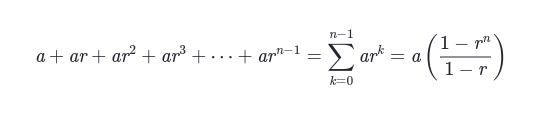Display mathematical expressions formatted as LaTeX.

Supported LaTeX functions are listed at https://katex.org/docs/supported.html.

Function signature[source]

st.latex(body, *, help=None)

Parameters

body (str or SymPy expression)

The string or SymPy expression to display as LaTeX. If str, it's a good idea to use raw Python strings since LaTeX uses backslashes a lot.

help (str)

An optional tooltip that gets displayed next to the LaTeX expression.

#### Example

import streamlit as st

st.latex(r'''
a + ar + a r^2 + a r^3 + \cdots + a r^{n-1} =
\sum_{k=0}^{n-1} ar^k =
a \left(\frac{1-r^{n}}{1-r}\right)
''')forum

### Still have questions?

Our forums are full of helpful information and Streamlit experts.